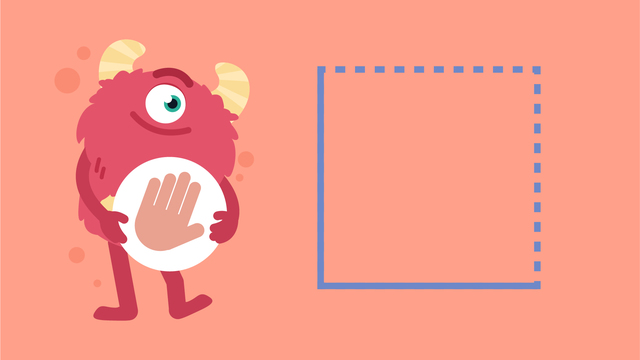# Drawing Shapes— Let's Practice!Rating

Ø 5.0 / 1 ratings
The authorsTeam Digital
Drawing Shapes— Let's Practice!
CCSS.MATH.CONTENT.K.G.B.5

## Basics on the topicDrawing Shapes— Let's Practice!

Let Razzi help you practice drawing shapes like triangles, squares, circles, and rectangles!

### TranscriptDrawing Shapes— Let's Practice!

Razzi says get a pencil and paper ready because today we're going to practice, “Drawing Shapes!” It's time to begin! Let's start by drawing a three-sided triangle! Razzi's triangle leans on the way down, then goes straight across, and leans on the way back up. Now try it on your paper! Did you make a triangle? Let's draw another shape! This time let's make a same sided square! Razzi's square goes straight down, straight across, back up and back across. Now it's your turn; try to make each side the same! Did you draw a square? Let's draw a round circle now! Razzi's circle starts at the top and curves all the way back around. Now it's your turn to try! How did your circle come out? Let's try one more: a four sided rectangle! Razzi's rectangle goes straight down a little bit, straight across a lot, back up a little, then back across a lot. Now it's your turn to try! Did you make a rectangle too? Razzi had so much fun practicing with you today! See you next time!

## Drawing Shapes— Let's Practice! exercise

Would you like to apply the knowledge you’ve learned? You can review and practice it with the tasks for the video Drawing Shapes— Let's Practice!.
• ### Name the shapes.

Hints

When Razzi drew a circle, it was round.

When Razzi drew a triangle, he drew three sides.

When Razzi drew a square, he made sure all four sides were the same length.

Solution

The image shows the correct name of each shape that Razzi drew.

• You can identify a triangle because it has three sides.
• You can identify a square because it has four equal sides.
• You can identify a circle because it is curved all the way around.
• You can identify a rectangle because it has four sides (two shorter sides and two longer sides).
• ### Tell how to draw shapes.

Hints

Practice tracing the circle with your finger. Do you move your finger in a straight line or a curved line?

Practice tracing the square with your finger. Are all of the sides the same length?

Practice tracing the rectangle with your finger. Are all of the sides the same length?

Practice tracing the triangle with your finger. Are all of the lines straight? Do any lines curve? Do any lines lean?

Solution

Here are the directions for drawing shapes. Get out your pencil and paper. Follow along and practice drawing each shape.

• A triangle leans on the way down, goes straight across, and leans on the way back up.
• A square goes straight down, straight across, back up, and back across. All of the sides are the same length.
• A circle starts at the top and curves all the way back around.
• A rectangle goes straight down a little bit, straight across a lot, back up a little, then back across a lot.
• ### Find shapes in real world objects.

Hints

A triangle is a shape with three sides. To draw it, lean on the way down, go straight across, and lean on the way back up.

A circle is a round shape. To draw it, start at the top and curve all the way back around.

A square is a shape with four equal sides. To draw it, go straight down, straight across, back up, and back across. Double check that all of your sides are equal length.

A rectangle is a shape with four sides. To draw it, go straight down a little bit, straight across a lot, back up a little, and then back across a lot.

Solution

Grab a pencil and paper. Try to draw your own shape train using only rectangles, squares, triangles, and circles.

What other things can you draw using these shapes? Try to also draw a house or a robot.

• ### How many sides do you need to draw each shape?

Hints

Touch the top of the circle. Trace all the way around. Did you make any straight line segments?

A circle does not have any straight sides.

Solution

To find the number of sides in a shape, you need to count each straight line.

• There are 3 sides in a triangle.
• There are 4 equal sides in a square.
• There are 4 sides in a rectangle.
• There are 0 sides in a circle.

• ### Identify each shape.

Hints

A circle is the only shape made with a curved line.

A triangle is the only shape that has lines that lean.

A square and a rectangle both have four, straight sides, but all of the sides are the same length on a square.

Solution

As shown in the image:

• the triangle is yellow
• the square is green
• the circle is blue
• the rectangle is violet

• ### Sort squares and rectangles.

Hints

To draw a square, Razzi drew 4 equal, straight sides that are all the same length.

To draw a rectangle, Razzi drew 2 longer sides and 2 shorter sides.

Solution

Both rectangles and squares have four straight sides.

All of the sides are the same length on a square. Two sides are shorter and two sides are longer on a rectangle.

• These objects are squares: a cracker, a window, a checker board, and a present.
• These objects are rectangles: a US flag, a dollar bill, a door, and a tablet.

Can you think of other objects that are squares or rectangles?